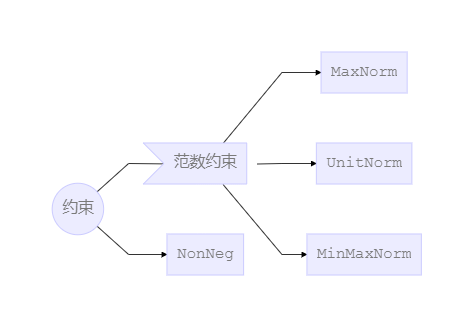null Keras 文档阅读笔记（不定期更新） - K码农

# Keras 文档阅读笔记（不定期更新）

## 模型

### Sequential 模型方法

• `compile`：用于配置模型的训练方法。
• `fit`：以固定数量的轮次（epoch）训练模型。
• `evaluate`：在测试模式，返回误差值和评估标准值，计算逐批次（batch）进行。
• `predict`：为输入样本生成输出预测，计算逐批次（batch）进行。
• `train_on_batch`：一批样品的单次梯度更新。
• `test_on_batch`：在一批样本上评估模型。
• `predict_on_batch`：返回一批样本的模型预测值。
• `fit_generator`：使用 Python 生成器或 `Sequence` 实例逐批生成的数据，按批次训练模型。生成器与模型并行运行，以提高效率。
• `evaluate_generator`：在数据生成器上评估模型。
• `predict_generator`：为来自数据生成器的输入样本生成预测。
• `get_layer`：根据名称（唯一）或索引值查找网络层。

## Model 类（函数式 API）

### 方法

• `compile`：用于配置模型的训练方法。
• `fit`：以固定数量的轮次（epoch）训练模型。
• `evaluate`：在测试模式，返回误差值和评估标准值，计算逐批次（batch）进行。
• `predict`：为输入样本生成输出预测，计算逐批次（batch）进行。
• `train_on_batch`：一批样品的单次梯度更新。
• `test_on_batch`：在一批样本上评估模型。
• `predict_on_batch`：返回一批样本的模型预测值。
• `fit_generator`：使用 Python 生成器或 `Sequence` 实例逐批生成的数据，按批次训练模型。生成器与模型并行运行，以提高效率。
• `evaluate_generator`：在数据生成器上评估模型。
• `predict_generator`：为来自数据生成器的输入样本生成预测。
• `get_layer`：根据名称（唯一）或索引值查找网络层。

## 层

### 关于 Keras 网络层

• `layer.get_weights()`：以含有 Numpy 矩阵的列表形式返回层的权重。
• `layer.set_weights(weights)`：从含有 Numpy 矩阵的列表中设置层的权重（与 `get_weights` 的输出形状相同）。
• `layer.get_config()`：返回包含层配置的字典。

• `layer.input`
• `layer.output`
• `layer.input_shape`
• `layer.output_shape`

• `layer.get_input_at(node_index)`
• `layer.get_output_at(node_index)`
• `layer.get_input_shape_at(node_index)`
• `layer.get_output_shape_at(node_index)`

### 核心层

• `Dense`

• `Activation`

• `Dropout`

• `Flatten`

• `Input`

`Input()` 用于实例化 Keras 张量。

• `Reshape`

• Permute

• `RepeatVector`

• `Lambda`

• `ActivityRegularization`

• `Masking`

• `SpatialDropout1D`

Dropout 的 Spatial 1D 版本。此版本的功能与 Dropout 相同，但它会丢弃整个 1D 的特征图而不是丢弃单个元素。

• `SpatialDropout2D`

Dropout 的 Spatial 2D 版本。此版本的功能与 Dropout 相同，但它会丢弃整个 2D 的特征图而不是丢弃单个元素。

• `SpatialDropout3D`

Dropout 的 Spatial 3D 版本。此版本的功能与 Dropout 相同，但它会丢弃整个 3D 的特征图而不是丢弃单个元素。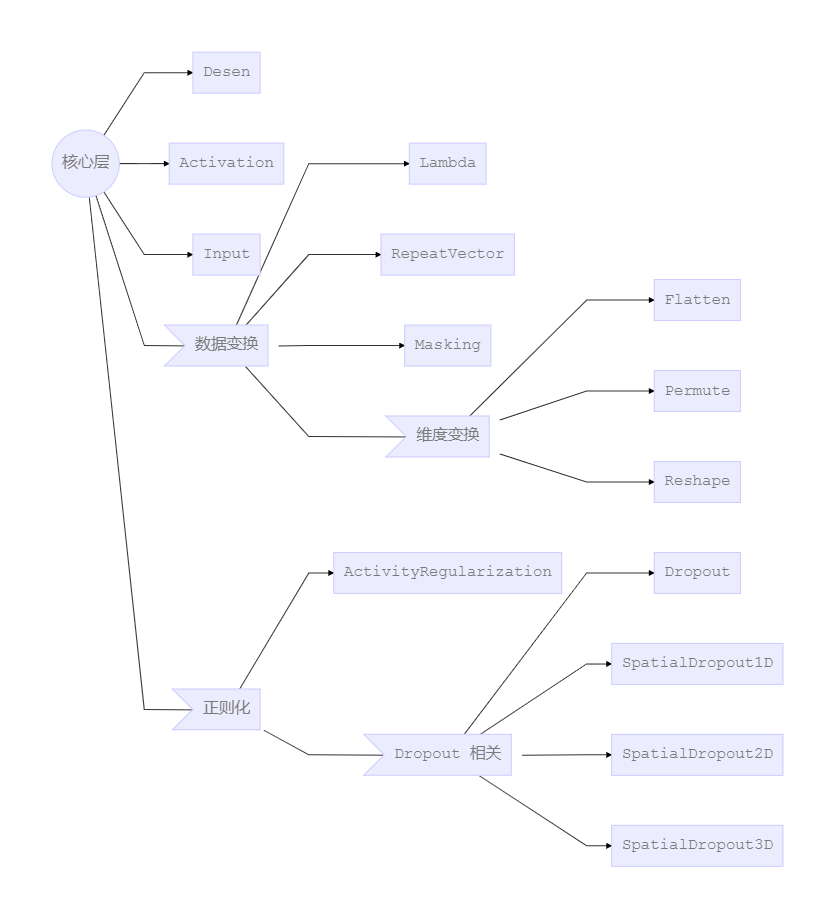### 卷积层

• `Conv1D`

1D 卷积层（例如时序卷积）。该层创建了一个卷积核，该卷积核以单个空间（或时间）维上的层输入进行卷积，以生成输出张量。

• `Conv2D`

2D 卷积层（例如对图像的空间卷积）。该层创建了一个卷积核，该卷积核对层输入进行卷积，以生成输出张量。

• `Conv3D`

3D 卷积层（例如立体空间卷积）。该层创建了一个卷积核，该卷积核对层输入进行卷积，以生成输出张量。

• `SeparableConv1D`

• `SeparableConv2D`

• `DepthwiseConv2D`

• `Conv2DTranspose`

• `Conv3DTranspose`

• `Cropping1D`

1D 输入的裁剪层（例如时间序列），它沿着时间维度（第 1 个轴）裁剪。

• `Cropping2D`

2D 输入的裁剪层（例如图像），它沿着空间维度裁剪，即宽度和高度。

• `Cropping3D`

3D 数据的裁剪层（例如空间或时空）。

• `UpSampling1D`

1D 输入的上采样层。

• `UpSampling2D`

2D 输入的上采样层。

• `UpSampling3D`

3D 输入的上采样层。

• `ZeroPadding1D`

1D 输入的零填充层（例如，时间序列）。

• `ZeroPadding2D`

2D 输入的零填充层（例如图像）。该图层可以在图像张量的顶部、底部、左侧和右侧添加零表示的行和列。

• `ZeroPadding3D`

3D 数据的零填充层（空间或时空）。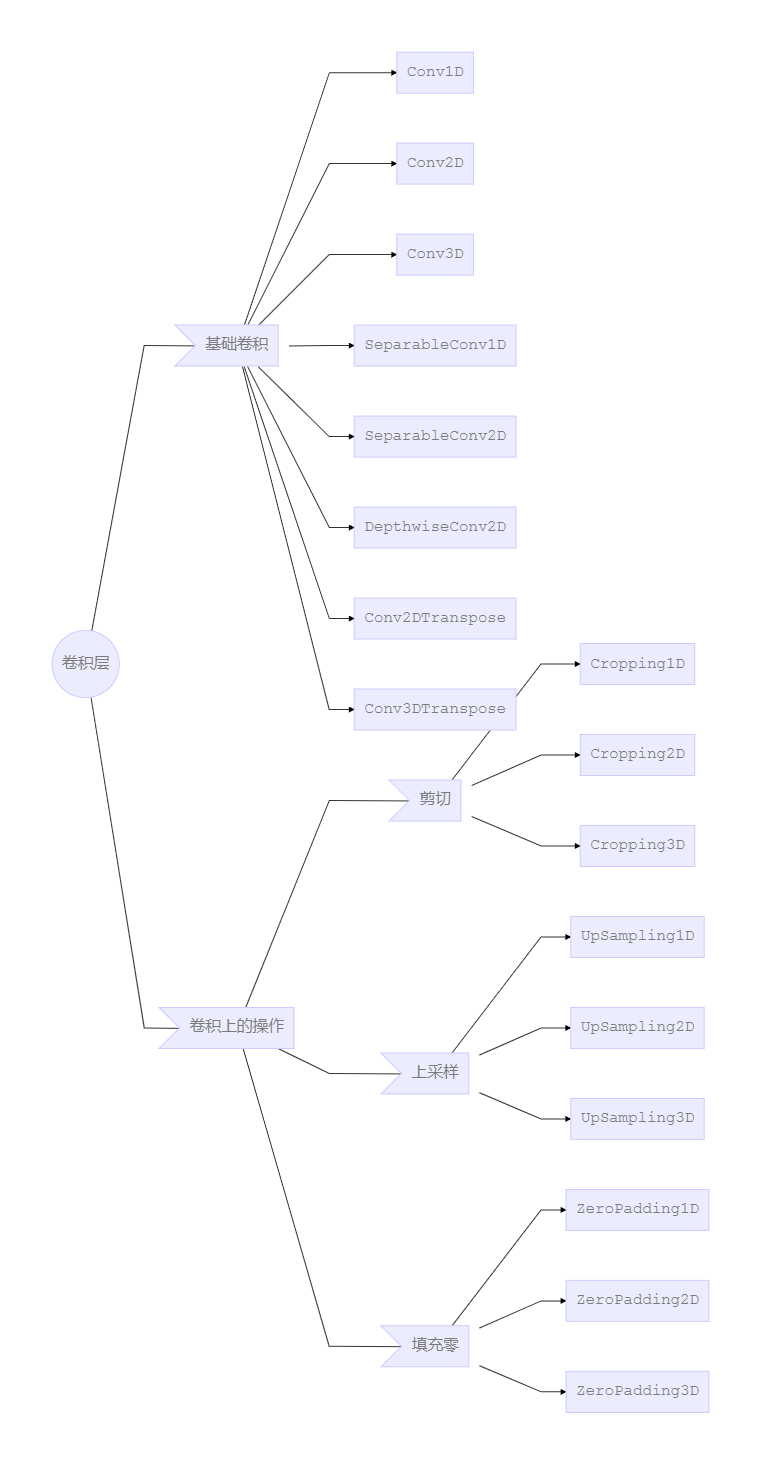### 池化层

• `MaxPooling1D`

• `MaxPooling2D`

• `MaxPooling3D`

• `AveragePooling1D`

• `AveragePooling2D`

• `AveragePooling3D`

• `GlobalMaxPooling1D`

• `GlobalAveragePooling1D`

• `GlobalMaxPooling2D`

• `GlobalAveragePooling2D`

• `GlobalMaxPooling3D`

• `GlobalAveragePooling3D`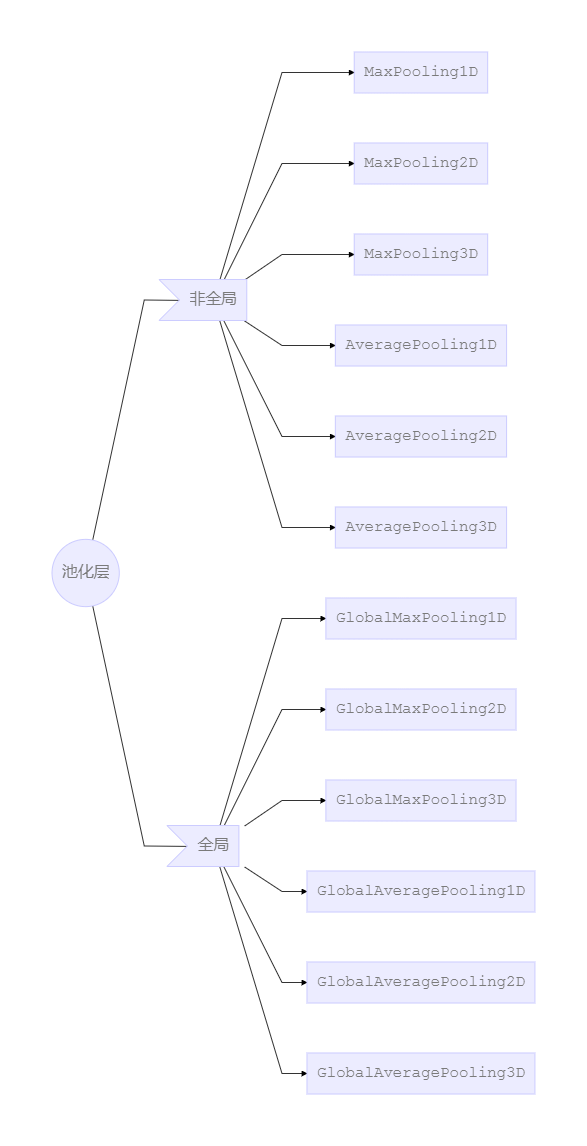### 循环层

• `RNN`

• `SimpleRNN`

• `GRU`

• `LSTM`

• `ConvLSTM2D`

• `ConvLSTM2DCell`

`ConvLSTM2D` 的单元（Cell）类。

• `SimpleRNNCell`

`SimpleRNN` 的单元（Cell）类。

• `GRUCell`

`GRU` 层的单元（Cell）类。

• LSTMCell

`LSTM` 层的单元（Cell）类。

• `CuDNNGRU`

CuDNN 支持的快速 GRU 实现。只能以 TensorFlow 后端运行在 GPU 上。

• `CuDNNLSTM`

CuDNN 支持的快速 LSTM 实现。只能以 TensorFlow 后端运行在 GPU 上。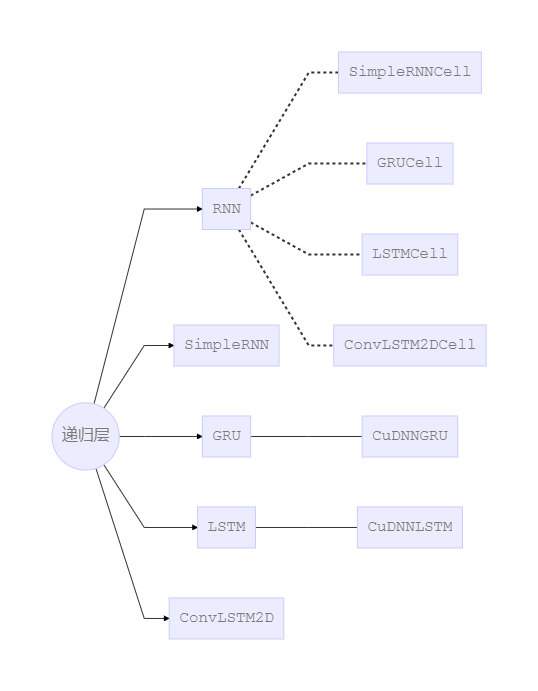### 融合层

• `Add`

• `Subtract`

• `Multiply`

• `Average`

• `Maximum`

• `Minimum`

• `Concatenate`

• `Dot`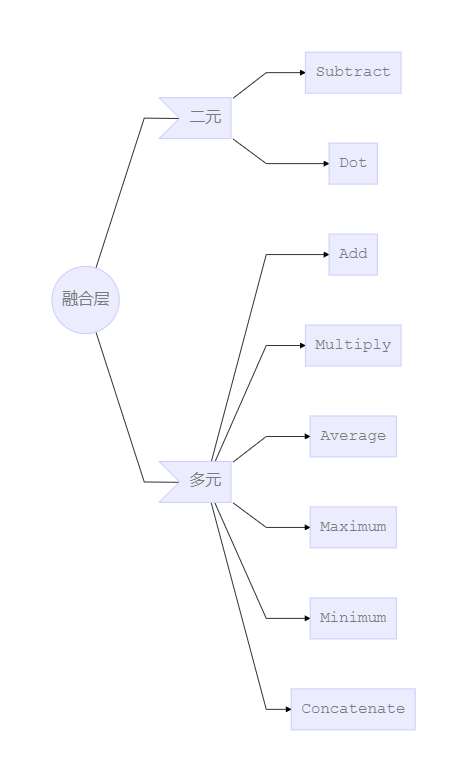### 高级激活层

• `LeakyReLU`

• `PReLU`

• `ELU`

• `ThresholdedReLU`

• `Softmax`

Softmax 激活函数。

• `ReLU`

ReLU 激活函数。使用默认值时，它返回逐个元素的 `max(x，0)`。否则：

• 如果 `x >= max_value`，返回 `f(x) = max_value`
• 如果 `threshold <= x < max_value`，返回 `f(x) = x`,
• 否则，返回 `f(x) = negative_slope * (x - threshold)`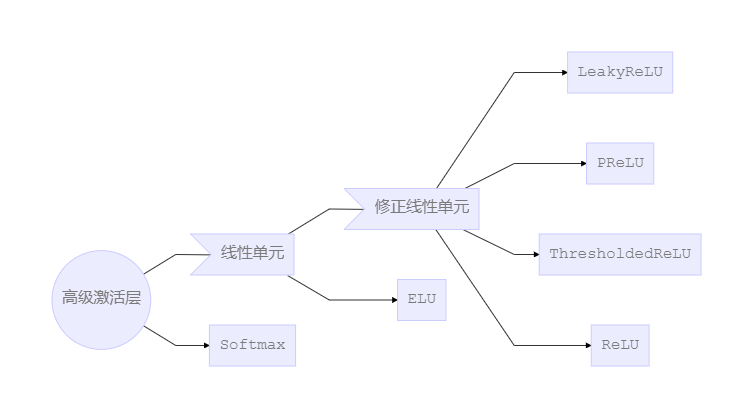### 其他层

#### 嵌入层

• `Embedding`

#### 标准化层

• `BatchNormalization`

#### 噪声层

• `GaussianNoise`

• `GaussianDropout`

• `AlphaDropout`

Alpha Dropout 是一种 Dropout，它保持输入的平均值和方差与原来的值不变，以确保即使在 dropout 后也能实现自我归一化。通过随机将激活设置为负饱和值，Alpha Dropout 非常适合按比例缩放的指数线性单元（SELU）。

#### 局部连接层

• `LocallyConnected1D`

1D 输入的局部连接层。

`LocallyConnected1D` 层与 `Conv1D` 层的工作方式相同，除了权值不共享外，也就是说，在输入的每个不同部分应用不同的一组过滤器。

• `LocallyConnected2D`

2D 输入的局部连接层。

`LocallyConnected2D` 层与 `Conv2D` 层的工作方式相同，除了权值不共享外，也就是说，在输入的每个不同部分应用不同的一组过滤器。

#### 层封装器

• `TimeDistributed`

• `Bidirectional`

`RNN` 的双向封装器，对序列进行前向和后向计算。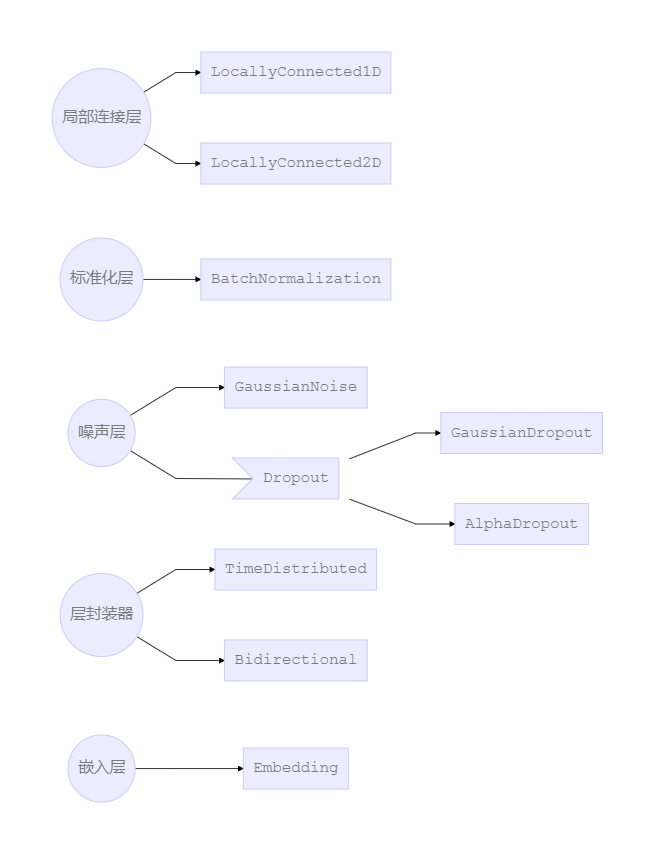## 损失函数

• `mean_squared_error`
• `mean_absolute_error`
• `mean_absolute_percentage_error`
• `mean_squared_logarithmic_error`
• `squared_hinge`
• `hinge`
• `categorical_hinge`
• `logcosh`

• `categorical_crossentropy`

• `sparse_categorical_crossentropy`
• `binary_crossentropy`
• `kullback_leibler_divergence`
• `poisson`
• `cosine_proximity`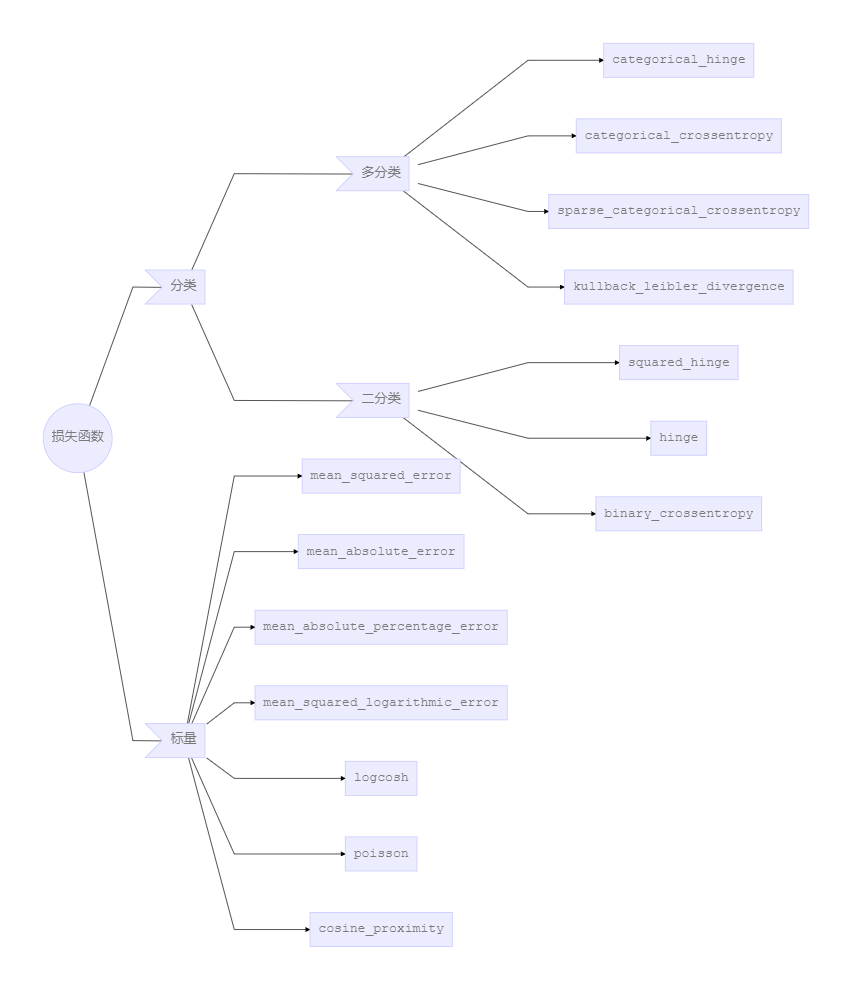## 评估标准

• `binary_accuracy`
• `categorical_accuracy`
• `sparse_categorical_accuracy`
• `top_k_categorical_accuracy`
• `sparse_top_k_categorical_accuracy`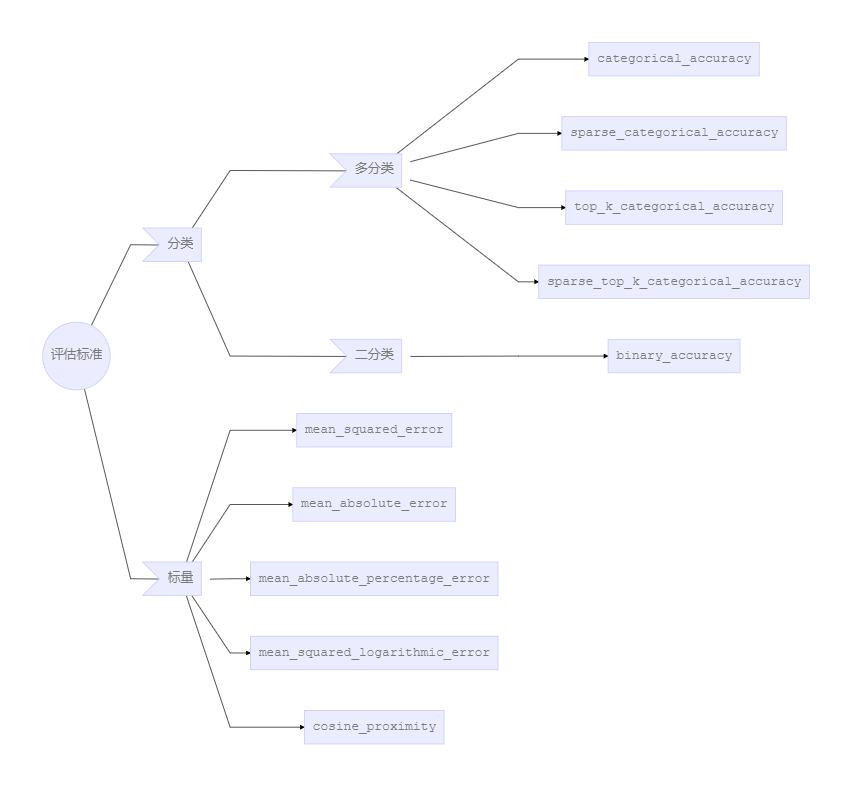## 优化器

• `SGD`

1. 动量（momentum）优化；
2. 学习率衰减（每次参数更新后）；
3. Nestrov 动量（NAG）优化。
• `RMSprop`

RMSProp 优化器，常是训练循环神经网络 RNN 的不错选择。建议使用优化器的默认参数（除了学习率 `lr`）。

• `Adagrad`

Adagrad 优化器，Adagrad 是一种具有特定参数学习率的优化器，它根据参数在训练期间的更新频率进行自适应调整。参数接收的更新越多，更新越小。建议使用优化器的默认参数。

• `Adadelta`

Adadelta 优化器，是 Adagrad 的一个具有更强鲁棒性的的扩展版本，可以设置初始学习速率和衰减因子。建议使用优化器的默认参数。

• `Adam`

Adam 本质上是 `RMSProp` 与动量 momentum 的结合

• `Adamax`

Adamax 优化器，是 Adam 算法基于无穷范数（infinity norm）的变种。

• `Nadam`

Nesterov 版本 `Adam` 优化器，Nadam 是采用 Nesterov momentum 版本的 `Adam` 优化器。建议使用优化器的默认参数。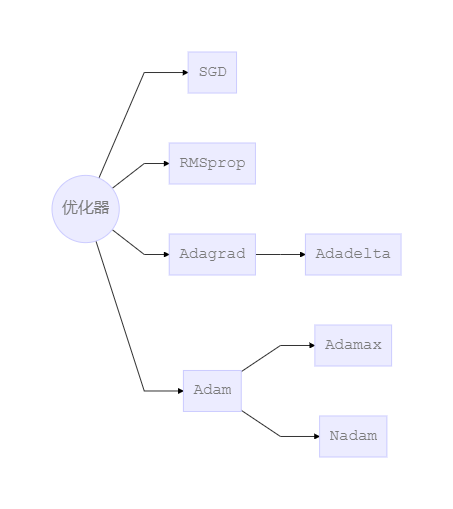## 激活函数

• `softmax`

Softmax 激活函数。

• `elu`

• `selu`

• `softplus`

Softplus 激活函数，返回 `log(exp(x) + 1)`.

• `softsign`

Softsign 激活函数，返回 `x / (abs(x) + 1)`.

• `relu`

• `tanh`

• `sigmoid`

Sigmoid 激活函数。

• `hard_sigmoid`

Hard sigmoid 激活函数，如果 `x < -2.5`，返回 `0`；如果 `x > 2.5`，返回 `1`；如果 `-2.5 <= x <= 2.5`，返回 `0.2 * x + 0.5`

• `exponential`

• `linear`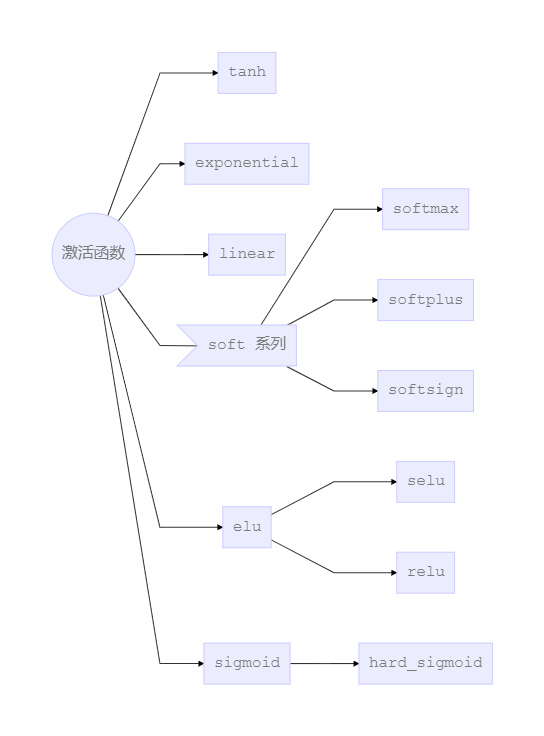## 正则化

• `kernel_regularizer``keras.regularizers.Regularizer` 的实例
• `bias_regularizer``keras.regularizers.Regularizer` 的实例
• `activity_regularizer``keras.regularizers.Regularizer` 的实例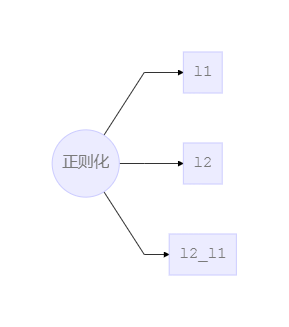## 约束

`constraints` 模块的函数允许在优化期间对网络参数设置约束（例如非负性）。

• `MaxNorm`

MaxNorm 最大范数权值约束。映射到每个隐藏单元的权值的约束，使其具有小于或等于期望值的范数。

• `NonNeg`

• `UnitNorm`

• `MinMaxNorm`

MinMaxNorm 最小/最大范数权值约束。映射到每个隐藏单元的权值的约束，使其范数在上下界之间。